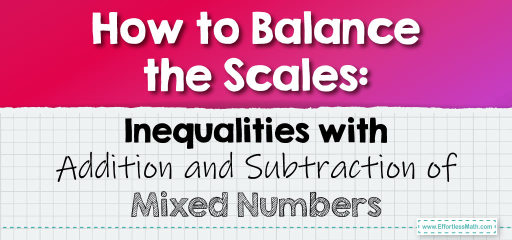# How to Balance the Scales: Inequalities with Addition and Subtraction of Mixed Numbers

Mixed numbers, which consist of a whole number and a fraction, often appear in real-world scenarios.When dealing with inequalities involving mixed numbers, understanding the impact of addition and subtraction is essential. In this guide, we’ll explore the intricacies of inequalities when adding or subtracting mixed numbers.

## Step-by-step Guide:

1. Basics of Inequalities:

Inequalities use symbols like $$<$$ (less than), $$>$$ (greater than), $$\leq$$ (less than or equal to), and $$\geq$$ (greater than or equal to) to compare two values.

2. Understanding Mixed Numbers:

A mixed number is a combination of a whole number and a fraction. For instance, $$2 \frac{1}{3}$$ is a mixed number.

3. Impact of Addition on Inequalities:

When you add the same mixed number to both sides of an inequality, the relationship between the two sides remains unchanged.

Example: If $$a > b$$, then $$a + c > b + c$$ for any mixed number $$c$$.

4. Impact of Subtraction on Inequalities:

Similarly, when you subtract the same mixed number from both sides of an inequality, the relationship remains unchanged.

Example: If $$a < b$$, then $$a – c < b – c$$ for any mixed number $$c$$.

5. Working with Mixed Numbers:

When adding or subtracting mixed numbers in inequalities:

– First, perform the operation on the whole numbers.

– Then, perform the operation on the fractions. If necessary, find a common denominator for the fractions.

### Example 1:

Given $$3 \frac{1}{4} < 4 \frac{1}{2}$$, what happens when we add $$1 \frac{3}{4}$$ to both sides?

Solution:

Adding $$1 \frac{3}{4}$$ to both sides:

$3 \frac{1}{4} + 1 \frac{3}{4} < 4 \frac{1}{2} + 1 \frac{3}{4}$

$5 < 6 \frac{1}{4}$

The Absolute Best Book for 5th Grade Students

### Example 2:

Given $$5 \frac{2}{3} > 4 \frac{1}{3}$$, what is the result when we subtract $$2 \frac{1}{3}$$ from both sides?

Solution:

Subtracting $$2 \frac{1}{3}$$ from both sides:

$5 \frac{2}{3} – 2 \frac{1}{3} > 4 \frac{1}{3} – 2 \frac{1}{3}$

$3 \frac{1}{3} > 2$

### Practice Questions:

1. If $$4 \frac{3}{5} > 3 \frac{2}{5}$$, what is the inequality after adding $$2 \frac{1}{5}$$ to both sides?

2. Given $$6 \frac{4}{7} < 7 \frac{3}{7}$$, what happens when we subtract $$3 \frac{2}{7}$$ from both sides?

3. If $$8 \frac{1}{8} > 7 \frac{3}{8}$$, what is the result when we add $$1 \frac{2}{8}$$ to both sides?

A Perfect Book for Grade 5 Math Word Problems!

1. $$6 \frac{4}{5} > 5 \frac{3}{5}$$

2. $$3 \frac{2}{7} < 4 \frac{1}{7}$$

3. $$9 \frac{3}{8} > 8 \frac{5}{8}$$

The Best Math Books for Elementary Students

### What people say about "How to Balance the Scales: Inequalities with Addition and Subtraction of Mixed Numbers - Effortless Math: We Help Students Learn to LOVE Mathematics"?

No one replied yet.

X
51% OFF

Limited time only!

Save Over 51%

SAVE $15 It was$29.99 now it is \$14.99